# The painter

In order for the painter to get the desired color, he must mix green and yellow in a ratio of 4: 7. If it has 28 l of green color, how many liters of yellow color should he add? How many liters of mixed color does he get?

y =  49 l
V =  77 l

### Step-by-step explanation: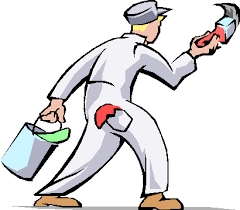Did you find an error or inaccuracy? Feel free to write us. Thank you!Tips to related online calculators
Check out our ratio calculator.
Do you have a linear equation or system of equations and looking for its solution? Or do you have a quadratic equation?

## Related math problems and questions:

• Orange colour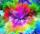In order for the painter to get orange, he has to mix yellow and red in a ratio of 3: 4. How many liters of orange did he mix if he used 8 liters of red?
• Eight blocks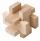Dana had the task to save the eight blocks of these rules: 1. Between two red cubes must be a different color. 2. Between two blue must be two different colors. 3. Between two green must be three different colors. 4. Between two yellow blocks must be four
• Solutions, mixturesHow many liters of 70% solution we must add to 5 liters of 30% solution to give us a 60% solution?
• Water solutionHow many grams of salt should we dissolve in 400 g of water to get a 20% solution?
• In and out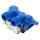The empty tank is filled in 12 minutes and empty in 16 minutes. How long does it take to fill if we forgot to close the drain? If the tank has 1000 l.
• Mixture 2How many liters of water must be added to 7 liters of a 20% solution to obtain a 10% solution?
• SugarsIn what ratio must two sorts of sugar, costing #390 and #315 per kg respectively, be mixed in order to produce a mixture worth #369 per kg?
• Hydrochloric acidDetermine the concentration of which must have a solution of hydrochloric acid that mixing 10 l of the solution with 8 liters of 26% solution to get the solution with a concentration of 50%?
• Vinegar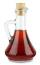What percentage vinegar we get if we mix 1 if dm³ eight percent vinegar with 1.5 dm³ six percent vinegar?
• Solutions, mixturesWe have 2 liters of 20% solution available. How much 70% solution do we need to add to it to get a 30% solution?
• Flags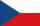How many different flags can be made from colors red, yellow, blue, green, white to consist of three different colors?
• Vinegar 2How many percentages of vinegar solution will we get if we mix 3.5 liters of 5.8% and 5 liters of 7.6% vinegar?
• Fertilizer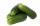Mr. Gherkin use for fertilization 7% solution of fertilizer. 17 liters of it still left. How much water must be added to the solution to make only 3% solution?
• Concentration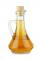How 0.5 liters of 8% vinegar diluted to a concentration of 20 hundredths % of vinegar? How many liters of water must be poured?
• Five pumps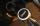Three same pumps fill the tank with 50400 liters of diesel in 7 hours. How many liters of diesel will it take in 4 hours if we add two more of the same pumps and pump them the same way? How much more (or less) will they get if we add 2 of the same pumps a
• Mixing waterWe should fill the 30-liter container with water at 60 degrees Celsius. How many liters of water 80 degrees C hot, and how many liters of water 20 degrees Celsius warm we have to mix?
• Solution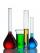In 469 dl red solution is 84 dl red color and in 102 dl blue solution is 52 dl blue color. How many dl of red and blue dl color solution must be mixed to get a mixture of 247 dl contain 116 dl of color?